# CVT Model

Symbols used: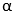= p, for primary pulley= s, for secondary pulley

 Radius Ratio [State]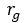Pulley’s Minimum Radius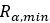Pulley’s inertia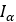Pulley’s Maximum Radius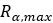Pulley’s Torque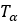Pulley’s Axial displacement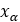Pulley’s Running radius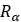Maximum Torque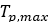Pulley’s RPM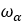Belt mass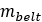Distance of flyweight from axis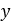Belt length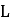Flyweight mass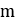Pulley’s distance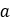Number of rollers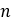Pulley’s Half angle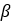Primary pulley Sheave angle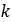Wrap angle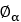Primary sheave ramp angle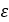Helix angle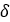Internal spring stiffness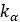Helix radius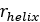Internal spring preloads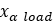Rotational Stiffness of secondary spring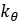Rotational spring preload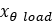Static Friction Coefficient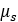Dynamic Friction Coefficient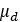CVT model evaluation happens in three steps:

## 1. Kinematic Evaluation

The input to primary pulley comes from engine RPM and are related as: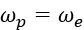The output of the secondary pulley is input to the gearbox and are related as: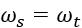The shift ratio of the CVT can be visualized in the illustration below:
At any time given the radius ratio and geometry information of pulleys and variator, we can find the running radii, wrap angles and axial displacements of the pulleys. The variator belt is assumed to be rigid.
The equations below are the geometric constraint in the model. After simplification, you can see that the final equation is implicit in terms of running radii and angle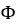therefore you can use the Newton Raphson method to solve for running radii and angle.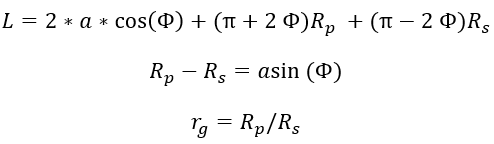Once you have running radii and angleyou can calculate the pulley’s axial displacements and wrap angles as follows: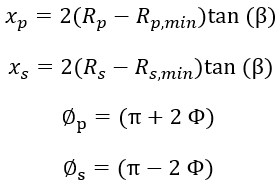The image below shows the variation of pulley radii, wrap angles andwith respect to radius ratio: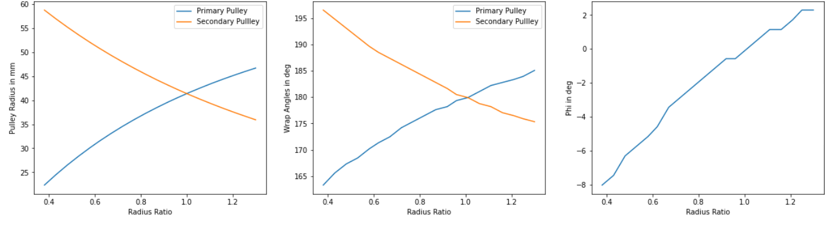## 2. Clamp For Calculation

The CVT model is set such that it can model mechanical, electric, and hydraulic CVT. By default, MS models a mechanical CVT based on following parameter in CVT property file.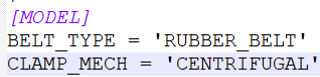If the CLAMP_MECH value is something other than ‘CENTRIFUGAL’ then its your responsibility to provide the clamping force values to the model. The clamp forces can be provided to the model using the solver variables shown in the image below:
Primary Pulley Clamping Force:
The ‘CENTRIFUGAL’ mechanism is used mostly in scooters where the clamping force is generated using the fly weights which rotates about the engine shaft and along the primary pulley sheave. The sheave has a ramp profile which converts the centrifugal force generated by flyweights into the clamping force.
Ramp Profile
The curve below shows the relation between the roller distance from shaft centreline (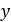) with axial shift of the sheave in primary pulley (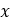).
The curve below shows the relation between the ramp angle (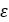) with the axial shift of the sheave in primary pulley ().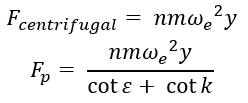Secondary Pulley Clamping Force:
The secondary pulley uses a torsional spring and a torque regulator cam also known as helix cam to generate the clamping force.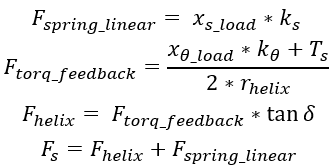## 3. Shift Ratio Calculation

To calculate the shift ratio, CMM model is used. According to the CMM model, the variator belt will shift if the clamp force ratio on the primary and secondary pulley is not equal to the stationary clamp force ratio. The stationary clamp force is the ratio of clamp force at which the belt is in equilibrium and does not shift.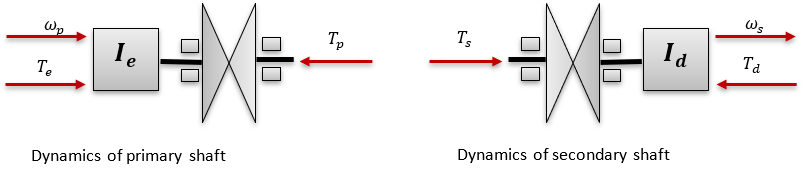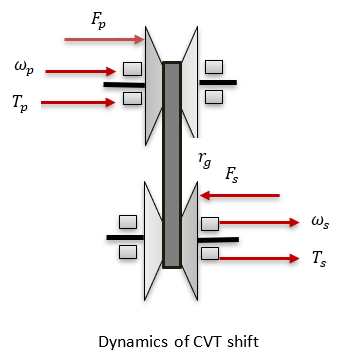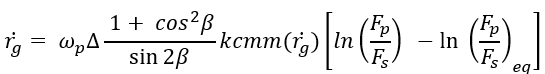The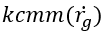coefficient needs to be measured experimentally.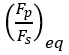is the stationary clamp force ratio.